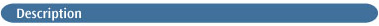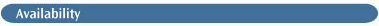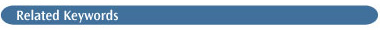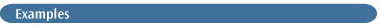# Density Functional (DFT) MethodsGaussian 03 offers a wide variety of Density Functional Theory (DFT) [75,76,448,449] models (see also [448,450,451,452,453,454,455,456,457,458,459,460,461] for discussions of DFT methods and applications). Energies , analytic gradients, and true analytic frequencies [197,198,199] are available for all DFT models. The same optimum memory sizes given by freqmem are recommended for the more general models.

The self-consistent reaction field (SCRF) can be used with DFT energies, optimizations, and frequency calculations to model systems in solution.

Pure DFT calculations will often want to take advantage of density fitting. See the discussion here for details.

The next subsection presents a very brief overview of the DFT approach. Following this, the specific functionals available in Gaussian 03 are given. The final subsection surveys considerations related to accuracy in DFT calculations.

Note: Polarizability derivatives (Raman intensities) and hyperpolarizabilities are not computed by default during DFT frequency calculations. Use Freq=Raman to request them.

### BACKGROUND

In Hartree-Fock theory, the energy has the form:

EHF = V + <hP> + 1/2<PJ(P)> - 1/2<PK(P)>

where the terms have the following meanings:

V                    The nuclear repulsion energy.

P                    The density matrix.

<hP>              The one-electron (kinetic plus potential) energy

1/2<PJ(P)>     The classical coulomb repulsion of the electrons.

-1/2<PK(P)>  The exchange energy resulting from the quantum (fermion) nature of electrons.

In density functional theory, the exact exchange (HF) for a single determinant is replaced by a more general expression, the exchange-correlation functional, which can include terms accounting for both exchange energy and the electron correlation which is omitted from Hartree-Fock theory:

EKS = V + <hP> + 1/2<PJ(P)> + EX[P] + EC[P]

where EX[P] is the exchange functional, and EC[P] is the correlation functional.

Hartree-Fock theory is really a special case of density functional theory, with EX[P] given by the exchange integral -1/2<PK(P)> and EC=0. The functionals normally used in density functional theory are integrals of some function of the density and possibly the density gradient:

EX[P] = ∫f(ρα(r),ρβ(r),∇ρα(r),∇ρβ(r))dr

where the methods differ in which function f is used for EX and which (if any) f is used for EC. In addition to pure DFT methods, Gaussian supports hybrid methods in which the exchange functional is a linear combination of the Hartree-Fock exchange and a functional integral of the above form. Proposed functionals lead to integrals which cannot be evaluated in closed form and are solved by numerical quadrature.

### KEYWORDS FOR DFT METHODS

Names for the various pure DFT models are given by combining the names for the exchange and correlation functionals. In some cases, standard synonyms used in the field are also available as keywords.

Exchange Functionals. The following exchange functionals are available in Gaussian 03:

• Slater: ρ4/3 with theoretical coefficient of 2/3, also referred to as Local Spin Density exchange [75,76,77].      Keyword: Used Alone: HFS, Comb. Form: S

• Xαρ4/3 with the empirical coefficient of 0.7, usually used when this exchange functional is used without a correlation functional [75,76,77]. Keyword: Used Alone: XAlpha, Comb. Form: XA.

• Becke 88: Becke's 1988 functional, which includes the Slater exchange along with corrections involving the gradient of the density . Keyword: Used Alone: HFB, Comb.Form: B.

• Perdew-Wang 91: The exchange component of Perdew and Wang's 1991 functional [463,464,465,466,467]. Keyword: Used Alone: N/A, Comb. Form: PW91.

• Barone's Modified PW91: The Perdew-Wang 1991 exchange functional as modified by Adamo and Barone . Keyword: Used Alone: N/A, Comb. Form: MPW.

• Gill 96: The 1996 exchange functional of Gill [469,470]. Keyword: Used Alone: N/A, Comb. Form: G96.

• PBE: The 1996 functional of Perdew, Burke and Ernzerhof [471,472]. Keyword: Used Alone: N/A, Comb. Form: PBE.

• OPTX: Handy's OPTX modification of Becke's exchange functional . Keyword: Used Alone: N/A, Comb. Form: O.

• TPSS: The exchange functional of Tao, Perdew, Staroverov, and Scuseria . Keyword: Used Alone: N/A, Comb. Form: TPSS.

The combination forms are used when one of these exchange functionals is used in combination with a correlation functional (see below).

Correlation Functionals. The following correlation functionals are available, listed by their corresponding keyword component:

• VWN: Vosko, Wilk, and Nusair 1980 correlation functional(III) fitting the RPA solution to the uniform electron gas, often referred to as Local Spin Density (LSD) correlation  (functional III in the paper).

• VWN V(VWN5): Functional V from the 1980 paper which fits the Ceperly-Alder solution to the uniform electron gas (this is the functional recommended in the paper) .

• LYP: The correlation functional of Lee, Yang, and Parr which includes both local and non-local terms [476,477].

• PL (Perdew Local): The local (non-gradient corrected) functional of Perdew (1981) .

• P86 (Perdew 86): The gradient corrections of Perdew, along with his 1981 local correlation functional .

• PW91 (Perdew/Wang 91): Perdew and Wang's 1991 gradient-corrected correlation functional [463,464,465,466,467].

• B95 (Becke 95): Becke's τ-dependent gradient-corrected correlation functional (defined as part of his one parameter hybrid functional .

• PBE: The 1996 gradient-corrected correlation functional of Perdew, Burke and Ernzerhof [471,472].

• TPSS: The τ-dependent gradient-corrected functional of Tao, Perdew, Staroverov, and Scuseria .

All of the keywords for these correlation functionals must be combined with the keyword for the desired exchange functional. For example, BLYP requests the Becke exchange functional and the LYP correlation functional. SVWN requests the Slater exchange and the VWN correlation functional, and is known in the literature by its synonym LSDA (Local Spin Density Approximation).

LSDA is a synonym for SVWN. Some other software packages with DFT facilities use the equivalent of SVWN5 when "LSDA" is requested. Check the documentation carefully for all packages when making comparisons.

Correlation Functional Variations. The following correlation functionals combine local and non-local terms from different correlation functionals:

• VP86: VWN5 local and P86 non-local correlation functional.

• V5LYP: VWN5 local and LYP non-local correlation functional.

Standalone Functionals. The following functionals are self-contained and are not combined with any other functional keyword components:

• VSXC: van Voorhis and Scuseria's τ-dependent gradient-corrected correlation functional .

• HCTH/*: Handy's family functional including gradient-corrected correlation [482,483,484]. HCTH refers to HCTH/407, HCTH93 to HCTH/93, HCTH147 to HCTH/147, and HCTH407 to HCTH/407. Note that the related HCTH/120 functional is not implemented.

Hybrid Functionals. Three hybrid functionals, which include a mixture of Hartree-Fock exchange with DFT exchange-correlation, are available via keywords:

• Becke Three Parameter Hybrid Functionals. These functionals have the form devised by Becke in 1993 :

A*EXSlater+(1-A)*EXHF+B*ΔEXBecke+ECVWN+C*ΔECnon-local

where A, B, and C are the constants determined by Becke via fitting to the G1 molecule set.

There are several variations of this hybrid functional. B3LYP uses the non-local correlation provided by the LYP expression, and VWN functional III for local correlation (not functional V). Note that since LYP includes both local and non-local terms, the correlation functional used is actually:

C*ECLYP+(1-C)*ECVWN

In other words, VWN is used to provide the excess local correlation required, since LYP contains a local term essentially equivalent to VWN.

B3P86 specifies the same functional with the non-local correlation provided by Perdew 86, and B3PW91 specifies this functional with the non-local correlation provided by Perdew/Wang 91.

• Becke One Parameter Hybrid Functionals. The B1B95 keyword is used to specify Becke's one-parameter hybrid functional as defined in the original paper .

The program also provides other, similar one parameter hybrid functionals, as implemented by Adamo and Barone [480,485]. In one variation, B1LYP, the LYP correlation functional is used (as described for B3LYP above). Another version, MPW1PW91, uses modified Perdew-Wang exchange and Perdew-Wang 91 correlation [468 ].

• Becke's 1998 revisions to B97 [486,487]. The keyword is B98, and it implements equation 2c in reference .

• Handy, Tozer and coworkers modification to B97: B971 .

• Wilson, Bradley and Tozer's modification to B97: B972 .

• The 1997 hybrid functional of Perdew, Burke and Ernzerhof . The keyword is PBE1PBE. This functional uses 25% exchange and 75% correlation weighting.

• O3LYP: A three-parameter functional similar to B3LYP:

A*EXLSD+(1-A)*EXHF+B*ΔEXOPTX+C*ΔECLYP+(1-C)ECVWN

where A, B and C are as defined by Cohen and Handy in reference .

• Half-and-half Functionals, which implement the following functionals:

BHandH:      0.5*EXHF + 0.5*EXLSDA + ECLYP

BHandHLYP:      0.5*EXHF + 0.5*EXLSDA + 0.5*ΔEXBecke88 + ECLYP

Note that these are not the same as the "half-and-half" functionals proposed by Becke (J. Chem. Phys. 98, 1372 (1993)). These functionals are included for backward-compatibility only.

• BMK: Boese and Martin's τ-dependent hybrid functional .

User-Defined Models. Gaussian 03 can use any model of the general form:

P2EXHF + P1(P4EXSlater + P3ΔExnon-local) + P6EClocal + P5ΔECnon-local

The only available local exchange method is Slater (S), which should be used when only local exchange is desired. Any combinable non-local exchange functional and combinable correlation functional may be used (as listed previously).

You specify the values of the six parameters with various non-standard options to the program:

• IOp(3/76=mmmmmnnnnn) sets P1 to mmmmm/10000 and P2 to nnnnn/10000. P1 is usually set to either 0.0 or 1.0, depending on whether an exchange functional is desired or not, and any scaling is accomplished using P3 and P4.

• IOp(3/77=mmmmmnnnnn) sets P3 to mmmmm/10000 and P4 to nnnnn/10000.

• IOp(3/78=mmmmmnnnnn) sets P5 to mmmmm/10000 and P6 to nnnnn/10000.

For example, IOp(3/76=1000005000) sets P1 to 1.0 and P2 to 0.5. Note that all values must be expressed using five digits, adding any necessary leading zeros.

Here is a route section specifying the functional corresponding to the B3LYP keyword:

`# BLYP IOp(3/76=1000002000) IOp(3/77=0720008000) IOp(3/78=0810010000) `

### ACCURACY CONSIDERATIONS

A DFT calculation adds an additional step to each major phase of a Hartree-Fock calculation. This step is a numerical integration of the functional (or various derivatives of the functional). Thus in addition to the sources of numerical error in Hartree-Fock calculations (integral accuracy, SCF convergence, CPHF convergence), the accuracy of DFT calculations also depends on number of points used in the numerical integration.

The "fine" integration grid (corresponding to Integral=FineGrid) is the default in Gaussian 03. This grid greatly enhances calculation accuracy at minimal additional cost. We do not recommend using any smaller grid in production DFT calculations. Note also that it is important to use the same grid for all calculations where you intend to compare energies (e.g., computing energy differences, heats of formation, and so on).

Larger grids are available when needed (e.g. tight optimization of certain kinds of systems). An alternate grid may be selected by including Integral=(Grid=N) in the route section (see the discussion of the Integral keyword for details).IOp, Int=Grid, Stable, TD, DenFitThe energy is reported in DFT calculations in a form similar to that of Hartree-Fock calculations. Here is the energy output from a B3LYP calculation:

`SCF Done:  E(RB+HF-LYP) =  -75.3197099428     A.U. after     5 cycles`

The item in parentheses following the E denotes the method used to obtain the energy. The output from a BLYP calculation is labeled similarly:

`SCF Done:  E(RB-LYP) =  -75.2867073414      A.U. after     5 cycles `